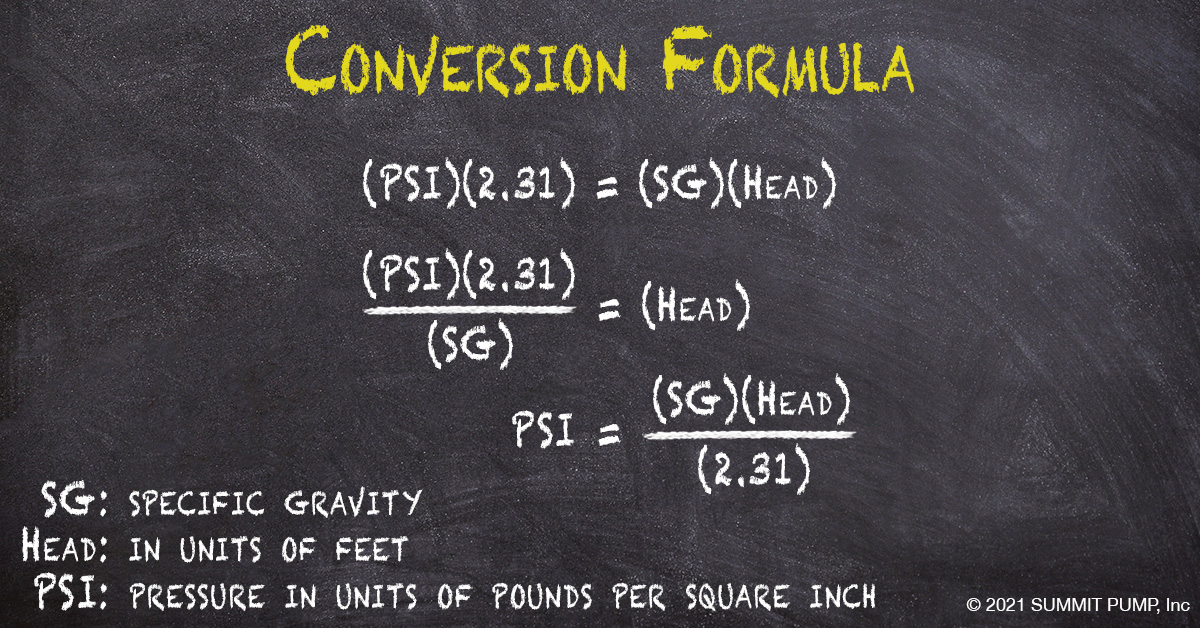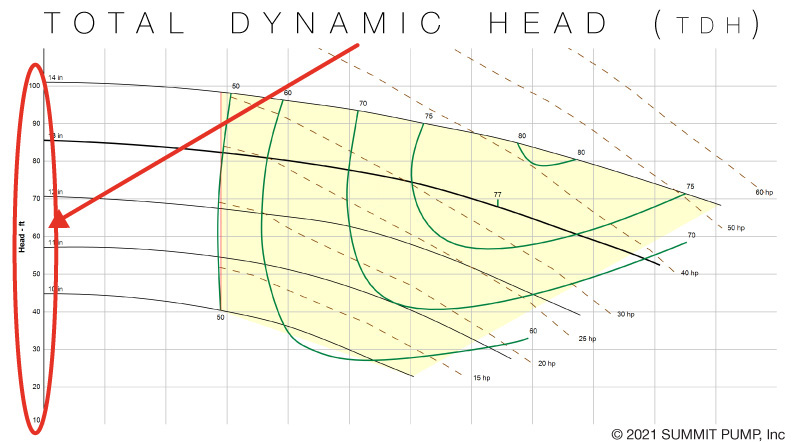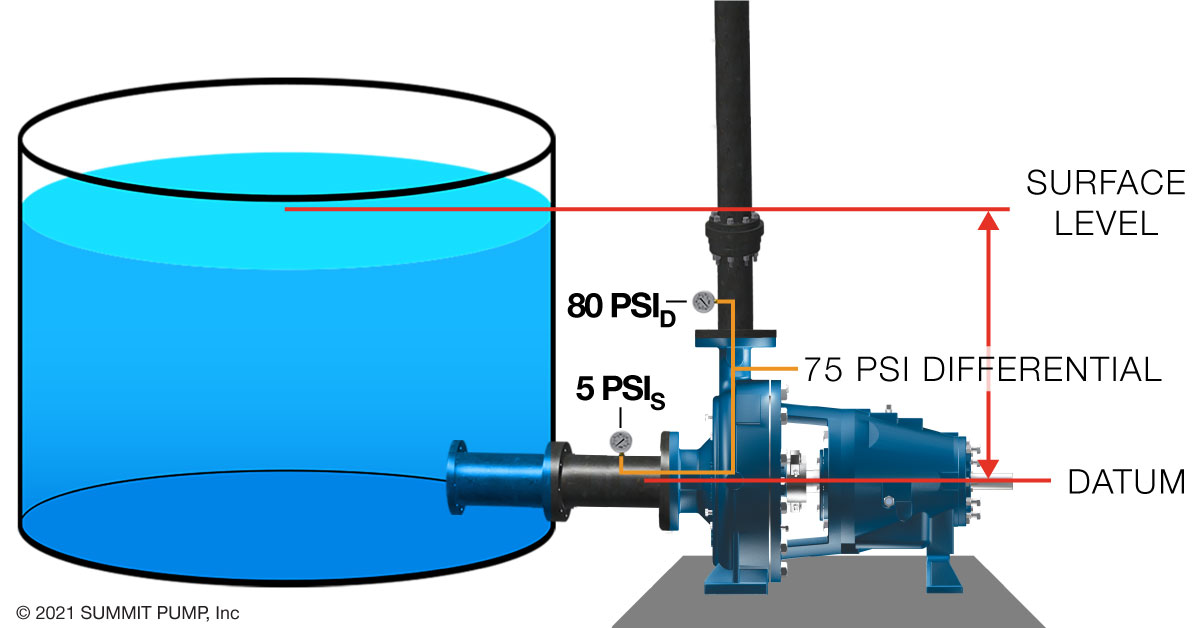1-800-489-8677 solutions@raross.com
Select PageUnderstanding your suction pressure and knowing how to read a vacuum is critical in identifying performance issues. In the field, instruments measure in units of PSI …whether gauge, absolute or vacuum. When diagnosing issues, PSI must be converted to head in order to calculate Total Dynamic Head (TDH).Consider Total Dynamic Head as the amount of energy the pump converts from suction to discharge. This information is critical in identifying where the pump operates on the performance curve.### Suction Pressure

To illustrate reading vacuum or pressure on the suction side of the pump, we will examine both a flooded suction and a suction lift application. Each example will assume the same pump, the same liquid and the same operation speed. The differential pressure of the pump will be the same for each example.

Note: For simplicity, we will not convert pressure readings to head in these examples, but understand the conversion mentioned above must be done in order to calculate TDH. For a more in-depth explanation, see my full article on the topic.

### Flooded Suction

In flooded suction, the liquid level is above the pump datum and has energy to provide to the pump. Meaning the pump does not need to convert this energy and will use this “given” energy elsewhere. In this case, a higher discharge pressure.Suction Lift

In Suction Lift, the pump must generate a low enough pressure at the eye of the impeller to have the fluid pushed into the pump by atmospheric pressure. Energy is used to make this happen and results in less energy available for pressure at the discharge.Notice the overall pressure readings are lower but the differential pressure (75 PSI) remains about the same in both flooded and suction lift applications (using the assumptions we made earlier).

Take-a-ways

Pressure gauges neglect velocity energy and read only one type of energy, which is pressure.Understanding your suction pressure and knowing how to read a vacuum is critical in calculating the pump’s TDH (Total Dynamic Head). Knowing the TDH can lead you to resolving several issues including operation cost, safety, pump life, inaccuracy or just nuisance issues.

-Jim Elsey